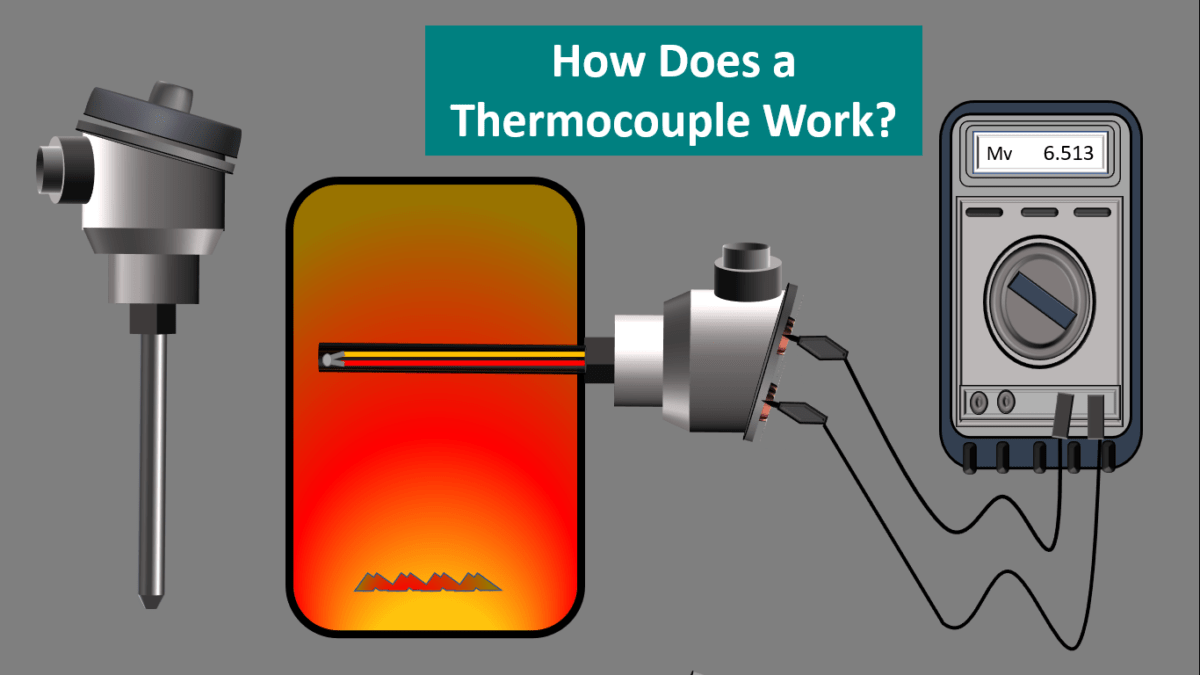# Thermocouple Basic Working Principle.Thermocouple is a kind of Temperature sensor that is used to measure the temperature at one specific point in the form of Emf or an Electric current.

Thermocouple can measure a wide range of Temperatures. It is a simple robust and cost effective temperature sensor used in various Industrial applications.

Working principle of Thermocouple

When two wires of Dissimilar metals are joined at both ends, two bimetallic junctions are formed.

One junction is heated using a heater and the other end is immersed in water.

When a multimeter is connected in the circuit, we can see that the multimeter is giving some reading.

This is the voltage between the hot and cold junctions

Now if we increase the temperature of the hot junction using heater we can see that the reading shown in the multimeter also increases, this proves that the voltage between these two junctions is the function of temperature difference between them this effect is known as SEEBECK EFFECT.

This phenomenon provides us with a simple way to electrically infer temperature. All thermocouple circuits no matter how simple or complex exhibit this fundamental property.

Construction of a Thermocouple.

A Thermocouple consists of two wire legs made from different metals.The Wire legs are welded together at one end Creating a Junction

The End of the junction is classified into three types namely

1.Ungrounded,

2.Grounded and

3.Exposed junction.

lets discuss these in detail,

1.Ungrounded junction:

In this type of junction the conductors are totally separated from the protecting cover The applications of this junction mainly include high pressure application works.

2.Grounded Junction:

In this type of junction the metal wires as well as the protection cover are connected together This type is used to measure the temperature in the acidic atmosphere  .

3.Exposed Junction:

The exposed junction is applicable in the areas where a QUICK response is required

This type of junction is used to measure the gas temperature

Working of a Thermocouple.

The junction is placed on the surface where we want to measure the temperature.

This junction is known as a Hot junction and the other end of the two wires are kept at a room temperature. This junction is known as Cold junction or Reference junction

According to the Seebeck Effect when the junction experiences a change in temperature a voltage is created.

A measuring instrument is connected in the circuit. The voltage measured by the measuring instrument is a function of temperature difference between the junctions

The voltage can then be interpreted using thermocouple reference tables to calculate the Temperature.

Hence by measuring the voltage we can calculate the temperature of the Hot junction

Do u want to know anything more about Instrumentation?